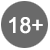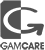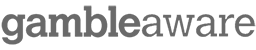# BankrollMob Forum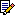BankrollMob Forum » Poker Strategies » How to calculate your winrate in cashgame ?

 How to calculate your winrate in cashgame ? 0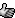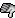Hi guys !

Here the question might looks like a bit noobish, but let me develop a lil' bit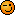So the basic question is how to calculate my winrate in cashgame. I started very recently and if remember well the articles i might had read a few time ago, they talk about bb/100. My deduction is that bb/100 is the number of big blinds you win per 100 hands (if it's not correct, please explain to me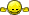).

More precisely, my question is how can i calculate this rate (i'd like to have a statistic idea of my game, but i'm not exactly sure to understand how to make it precisely).
I know trackers exist, but for now i have not enough money to afford one. And i'm playing at a lobby that is not tracked by ranking sites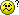So if u have any idea how to compute this precisely (usually, i do it manually with an excel sheet for the sng's for example).

Thanks for ur help guys+1Without trackers you have to do it the hard way and write down how many hands you played in a session. Then look at your bankroll at the start and the end of the session and do some basic math.

And the BB in BB/100 is big bets (2x big blind), as this was developed for fixed limit games.

 0thanks mate, its very helpfulI think i should be able to differentiate my winnings from cashgames and those from sng's with the rating sites for sng's. and since i have the number of hands registered, it should be makable to calculate this BB/100.

Any idea of what should possibly this BB/100 in low stakes cash game (0.02/0.04\$ blinds) for a winning player ?

 0Everything above 0 is good.A winrate of 3-5 should be doable.

 0Good question! If there is a rough calculation that can be used other then the use of a Tracker please advise. I mean I understand the basis using BB/100, like you mentioned to calculate your overall win rate wouldn't you have to find the average of your paid blinds from all games played? I mean if I play 3 SnG's @ \$1.10 and 3 tournies with various by-in amounts wouldn't I have to calculate my average by-in then take my BB/100. Whatever that number would be equal to would then need divided by my average by-in or am I just all messed up here.
Or, are SnG's and tourneies calculated using two different methods? Sorry it sounds so confusing!

 0I haven't head of that statistic before, but I play mainly SnG's so it's not to hard to manually track ROI. Could you track your ROI just by noting how much you sit and leave each table with? Then divide the sum of the two numbers? Or would you have to track each bet? Tedious for sure.

 0I figure mahdrof, Islandjack, doz01 or B1gfoot could help answer these questions. When it comes to serious poker questions / advice they've always provided good input. I'd like to find out a bit about this myself mate! Good Luck to All!

 0Posted by Greenmohave:Good question! If there is a rough calculation that can be used other then the use of a Tracker please advise. I mean I understand the basis using BB/100, like you mentioned to calculate your overall win rate wouldn't you have to find the average of your paid blinds from all games played? I mean if I play 3 SnG's @ \$1.10 and 3 tournies with various by-in amounts wouldn't I have to calculate my average by-in then take my BB/100. Whatever that number would be equal to would then need divided by my average by-in or am I just all messed up here. Or, are SnG's and tourneies calculated using two different methods? Sorry it sounds so confusing!

Hehe Greenmohave, i think u made a lil bit of confusion here. Talking about BB/100 is when you are playing cash game (ring games), not sng's. For sng's the calculation is mostly based on the ROI (you dont look the number of hand, but the number of sng's played : ROI = [Profit (or loss) - total buy in]/total buy in).

Here is how i computed my BB/100 (you do this with ring games, not sng's !!!!!) :
P = Total profit/loss
H = number of hands (related to this profit)
BB = 2*big blinds = 2*0.04 = 0.08 (since i play at the 0.02/0.04\$ limit)

I first calculated my profit/100 hands :
P/(H/100)

Then i calculated my BB/100
[ P/(H/100) ]/0.08

(If someone see something wrong in my calculation, plz tell me, i'm here to learn, thank you)

 0A very simple thing to do if you don't have the means to track your hands, though less accurate because there are external factors that may change a little bit the numbers, is to calculate \$/hour. Just write down the length of the session and how much \$ you won/lost. Of course you have to take into account the limit and type of table you are playing on.

 0Posted by Tchungpo:
 Posted by Greenmohave:Good question! If there is a rough calculation that can be used other then the use of a Tracker please advise. I mean I understand the basis using BB/100, like you mentioned to calculate your overall win rate wouldn't you have to find the average of your paid blinds from all games played? I mean if I play 3 SnG's @ \$1.10 and 3 tournies with various by-in amounts wouldn't I have to calculate my average by-in then take my BB/100. Whatever that number would be equal to would then need divided by my average by-in or am I just all messed up here. Or, are SnG's and tourneies calculated using two different methods? Sorry it sounds so confusing!

Hehe Greenmohave, i think u made a lil bit of confusion here. Talking about BB/100 is when you are playing cash game (ring games), not sng's. For sng's the calculation is mostly based on the ROI (you dont look the number of hand, but the number of sng's played : ROI = [Profit (or loss) - total buy in]/total buy in).

Here is how i computed my BB/100 (you do this with ring games, not sng's !!!!!) :
P = Total profit/loss
H = number of hands (related to this profit)
BB = 2*big blinds = 2*0.04 = 0.08 (since i play at the 0.02/0.04\$ limit)

I first calculated my profit/100 hands :
P/(H/100)

Then i calculated my BB/100
[ P/(H/100) ]/0.08

(If someone see something wrong in my calculation, plz tell me, i'm here to learn, thank you)

how i do:

P/bb = number of bb you win/lose. Then ( )/100 and you have it.

The first thing you must do when you have enough \$\$ is buy HM or PT3. Excellent soft to maximize winnings and for analyze our game/wins/loss etc...BankrollMob Forum » Poker Strategies » How to calculate your winrate in cashgame ?

Forum Rules | Support & FAQ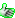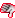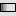Please Play Responsibly